# NCERT solutions for Physics Part 1 and 2 Class 12 chapter 9 - Ray Optics and Optical Instruments [Latest edition]

#### Chapters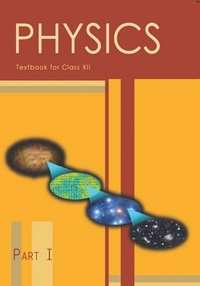## Chapter 9: Ray Optics and Optical Instruments

Exercise
Exercise [Pages 344 - 348]

### NCERT solutions for Physics Part 1 and 2 Class 12 Chapter 9 Ray Optics and Optical Instruments Exercise [Pages 344 - 348]

Exercise | Q 9.1 | Page 344

A small candle, 2.5 cm in size is placed at 27 cm in front of a concave mirror of radius of curvature 36 cm. At what distance from the mirror should a screen be placed in order to obtain a sharp image? Describe the nature and size of the image. If the candle is moved closer to the mirror, how would the screen have to be moved?

Exercise | Q 9.2 | Page 344

A 4.5 cm needle is placed 12 cm away from a convex mirror of focal length 15 cm. Give the location of the image and the magnification. Describe what happens as the needle is moved farther from the mirror.

Exercise | Q 9.3 | Page 344

A tank is filled with water to a height of 12.5 cm. The apparent depth of a needle lying at the bottom of the tank is measured by a microscope to be 9.4 cm. What is the refractive index of water? If water is replaced by a liquid of refractive index 1.63 up to the same height, by what distance would the microscope have to be moved to focus on the needle again?

Exercise | Q 9.4 | Page 344

Figures (a) and (b) show the refraction of a ray in air incident at 60° with the normal to a glass-air and water-air interface, respectively. Predict the angle of refraction in the glass when the angle of incidence in water is 45° with the normal to a water-glass interface [Figure (c)].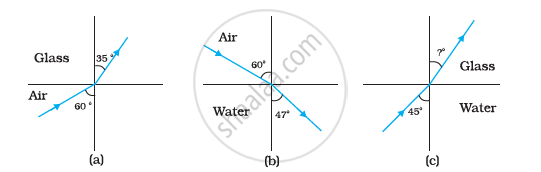Exercise | Q 9.5 | Page 344

A small bulb is placed at the bottom of a tank containing water to a depth of 80 cm. What is the area of the surface of water through which light from the bulb can emerge out? Refractive index of water is 1.33. (Consider the bulb to be a point source.)

Exercise | Q 9.6 | Page 344

A prism is made of glass of unknown refractive index. A parallel beam of light is incident on the face of the prism. The angle of minimum deviation is measured to be 40°. What is the refractive index of the material of the prism? The refracting angle of the prism is 60°. If the prism is placed in water (refractive index 1.33), predict the new angle of minimum deviation of a parallel beam of light.

Exercise | Q 9.7 | Page 344

Double-convex lenses are to be manufactured from a glass of refractive index 1.55, with both faces of the same radius of curvature. What is the radius of curvature required if the focal length is to be 20 cm?

Exercise | Q 9.8 | Page 344

A beam of light converges at a point P. Now a lens is placed in the path of the convergent beam 12 cm from P. At what point does the beam converge if the lens is (a) a convex lens of focal length 20 cm, and (b) a concave lens of focal length 16 cm?

Exercise | Q 9.9 | Page 344

An object of size 3.0 cm is placed 14 cm in front of a concave lens of focal length 21 cm. Describe the image produced by the lens. What happens if the object is moved further away from the lens?

Exercise | Q 9.10 | Page 345

What is the focal length of a convex lens of focal length 30 cm in contact with a concave lens of focal length 20 cm? Is the system a converging or a diverging lens? Ignore thickness of the lenses.

Exercise | Q 9.11 | Page 345

A compound microscope consists of an objective lens of focal length 2.0 cm and an eyepiece of focal length 6.25 cm separated by a distance of 15 cm. How far from the objective should an object be placed in order to obtain the final image at (a) the least distance of distinct vision (25 cm), and (b) at infinity? What is the magnifying power of the microscope in each case?

Exercise | Q 9.12 | Page 345

A person with a normal near point (25 cm) using a compound microscope with the objective of focal length 8.0 mm and an eyepiece of focal length 2.5 cm can bring an object placed at 9.0 mm from the objective in sharp focus. What is the separation between the two lenses? Calculate the magnifying power of the microscope.

Exercise | Q 9.13 | Page 345

A small telescope has an objective lens of focal length 144 cm and an eyepiece of focal length 6.0 cm. What is the magnifying power of the telescope? What is the separation between the objective and the eyepiece?

Exercise | Q 9.14 | Page 345

(a) A giant refracting telescope at an observatory has an objective lens of focal length 15 m. If an eyepiece of focal length 1.0 cm is used, what is the angular magnification of the telescope?

(b) If this telescope is used to view the moon, what is the diameter of the image of the moon formed by the objective lens? The diameter of the moon is 3.48 × 106 m, and the radius of lunar orbit is 3.8 × 108 m.

Exercise | Q 9.15 (a) | Page 345

Use the mirror equation to show that an object placed between f and 2f of a concave mirror produces a real image beyond 2f.

Exercise | Q 9.15 (b) | Page 345

Use mirror equation to show that a convex mirror always produces a virtual image independent of the location of the object.

Exercise | Q 9.15 (c) | Page 345

Use the mirror equation to deduce that the virtual image produced by a convex mirror is always diminished in size and is located between the focus and the pole.

Exercise | Q 9.15 (d) | Page 345

Use the mirror equation to deduce that an object placed between the pole and focus of a concave mirror produces a virtual and enlarged image.

Exercise | Q 9.16 | Page 345

A small pin fixed on a table top is viewed from above from a distance of 50 cm. By what distance would the pin appear to be raised if it is viewed from the same point through a 15 cm thick glass slab held parallel to the table? Refractive index of glass = 1.5. Does the answer depend on the location of the slab?

Exercise | Q 9.17 | Page 345

(a) Figure shows a cross-section of a ‘light pipe’ made of a glass fibre of refractive index 1.68. The outer covering of the pipe is made of a material of refractive index 1.44. What is the range of the angles of the incident rays with the axis of the pipe for which total reflections inside the pipe take place, as shown in the figure?

(b) What is the answer if there is no outer covering of the pipe?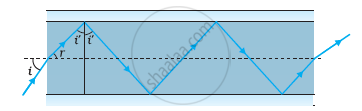Exercise | Q 9.18 (a) | Page 346

You have learnt that plane and convex mirrors produce virtual images of objects. Can they produce real images under some circumstances? Explain.

Exercise | Q 9.18 (b) | Page 346

A virtual image, we always say, cannot be caught on a screen. Yet when we ‘see’ a virtual image, we are obviously bringing it on to the ‘screen’ (i.e., the retina) of our eye. Is there a contradiction?

Exercise | Q 9.18 (c) | Page 346

A diver under water, looks obliquely at a fisherman standing on the bank of a lake. Would the fisherman look taller or shorter to the diver than what he actually is?

Exercise | Q 9.18 (d) | Page 346

Does the apparent depth of a tank of water change if viewed obliquely? If so, does the apparent depth increase or decrease?

Exercise | Q 9.18 (e) | Page 346

The refractive index of diamond is much greater than that of ordinary glass. Is this fact of some use to a diamond cutter?

Exercise | Q 9.19 | Page 346

The image of a small electric bulb fixed on the wall of a room is to be obtained on the opposite wall 3 m away by means of a large convex lens. What is the maximum possible focal length of the lens required for the purpose?

Exercise | Q 9.20 | Page 346

A screen is placed 90 cm from an object. The image of the object on the screen is formed by a convex lens at two different locations separated by 20 cm. Determine the focal length of the lens.

Exercise | Q 9.21 | Page 346

(a) Determine the ‘effective focal length’ of the combination of the two lenses, if they are placed 8.0 cm apart with their principal axes coincident. Does the answer depend on which side of the combination a beam of parallel light is incident? Is the notion of the effective focal length of this system useful at all?

(b) An object 1.5 cm in size is placed on the side of the convex lens in the arrangement (a) above. The distance between the object and the convex lens is 40 cm. Determine the magnification produced by the two-lens system and the size of the image.

Exercise | Q 9.22 | Page 346

At what angle should a ray of light be incident on the face of a prism of refracting angle 60° so that it just suffers total internal reflection at the other face? The refractive index of the material of the prism is 1.524.

Exercise | Q 9.23 | Page 346

A card sheet divided into squares each of size 1 mm2 is being viewed at a distance of 9 cm through a magnifying glass (a converging lens of focal length 9 cm) held close to the eye.

(a) What is the magnification produced by the lens? How much is the area of each square in the virtual image?

(b) What is the angular magnification (magnifying power) of the lens?

(c) Is the magnification in (a) equal to the magnifying power in (b)? Explain.

Exercise | Q 9.24 | Page 347

(a) At what distance should the lens be held from the figure in order to view the squares distinctly with the maximum possible magnifying power?

(b) What is the magnification in this case?

(c) Is the magnification equal to the magnifying power in this case? Explain.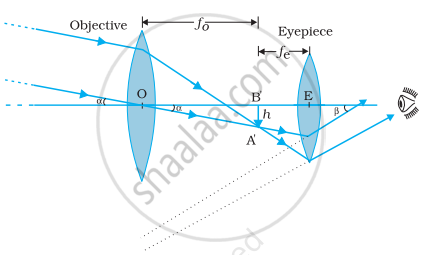Exercise | Q 9.25 | Page 347

What should be the distance between the object and the magnifying glass if the virtual image of each square in the figure is to have an area of 6.25 mm2. Would you be able to see the squares distinctly with your eyes very close to the magnifier?

Exercise | Q 9.26 (a) | Page 347

The angle subtended at the eye by an object is equal to the angle subtended at the eye by the virtual image produced by a magnifying glass. In what sense then does a magnifying glass provide angular magnification?

Exercise | Q 9.26 (b) | Page 347

In viewing through a magnifying glass, one usually positions one’s eyes very close to the lens. Does angular magnification change if the eye is moved back?

Exercise | Q 9.26 (c) | Page 347

Magnifying power of a simple microscope is inversely proportional to the focal length of the lens. What then stops us from using a convex lens of smaller and smaller focal length and achieving greater and greater magnifying power?

Exercise | Q 9.26 (d) | Page 347

Why must both the objective and the eyepiece of a compound microscope have short focal lengths?

Exercise | Q 9.26 (e) | Page 347

When viewing through a compound microscope, our eyes should be positioned not on the eyepiece but a short distance away from it for best viewing. Why? How much should be that short distance between the eye and eyepiece?

Exercise | Q 9.27 | Page 347

An angular magnification (magnifying power) of 30X is desired using an objective of focal length 1.25 cm and an eyepiece of focal length 5 cm. How will you set up the compound microscope?

Exercise | Q 9.28 | Page 347

A small telescope has an objective lens of focal length 140 cm and an eyepiece of focal length 5.0 cm. What is the magnifying power of the telescope for viewing distant objects when

(a) the telescope is in normal adjustment (i.e., when the final image is at infinity)?

(b) the final image is formed at the least distance of distinct vision (25 cm)?

Exercise | Q 9.29 (a) | Page 347

For the telescope is in normal adjustment (i.e., when the final image is at infinity)? what is the separation between the objective lens and the eyepiece?

Exercise | Q 9.29 (b) | Page 347

If this telescope is used to view a 100 m tall tower 3 km away, what is the height of the image of the tower formed by the objective lens?

Exercise | Q 9.29 (c) | Page 348

What is the height of the final image of the tower if it is formed at 25 cm?

Exercise | Q 9.30 | Page 348

A Cassegrain telescope uses two mirrors as shown in the figure. Such a telescope is built with the mirrors 20 mm apart. If the radius of curvature of the large mirror is 220 mm and the small mirror is 140 mm, where will the final image of an object at infinity be?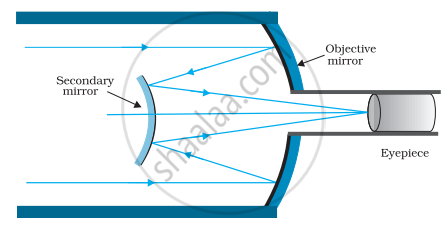Exercise | Q 9.31 | Page 348

Light incident normally on a plane mirror attached to a galvanometer coil retraces backward as shown in Figure. A current in the coil produces a deflection of 3.5° of the mirror. What is the displacement of the reflected spot of light on a screen placed 1.5 m away?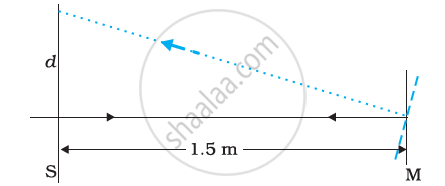Exercise | Q 9.32 | Page 348

Figure shows an equiconvex lens (of refractive index 1.50) in contact with a liquid layer on top of a plane mirror. A small needle with its tip on the principal axis is moved along the axis until its inverted image is found at the position of the needle. The distance of the needle from the lens is measured to be 45.0 cm. The liquid is removed and the experiment is repeated. The new distance is measured to be 30.0 cm. What is the refractive index of the liquid?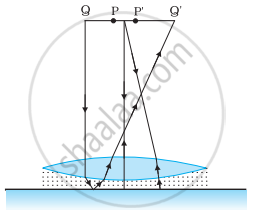## Chapter 9: Ray Optics and Optical Instruments

Exercise## NCERT solutions for Physics Part 1 and 2 Class 12 chapter 9 - Ray Optics and Optical Instruments

NCERT solutions for Physics Part 1 and 2 Class 12 chapter 9 (Ray Optics and Optical Instruments) include all questions with solution and detail explanation. This will clear students doubts about any question and improve application skills while preparing for board exams. The detailed, step-by-step solutions will help you understand the concepts better and clear your confusions, if any. Shaalaa.com has the CBSE Physics Part 1 and 2 Class 12 solutions in a manner that help students grasp basic concepts better and faster.

Further, we at Shaalaa.com provide such solutions so that students can prepare for written exams. NCERT textbook solutions can be a core help for self-study and acts as a perfect self-help guidance for students.

Concepts covered in Physics Part 1 and 2 Class 12 chapter 9 Ray Optics and Optical Instruments are Total Internal Reflection, Refraction, Ray Optics - Mirror Formula, Reflection of Light by Spherical Mirrors, Telescope, The Eye, Snell’s Law, Concave Mirror, Rarer and Denser Medium, Lensmaker's Formula, Power of a Lens, Magnification, Refraction at Spherical Surfaces, Light Process and Photometry, Refraction Through a Prism, Refraction at Spherical Surfaces and Lenses, Refraction by a Lens, Thin Lens Formula, Lenses, The Microscope, Some Natural Phenomena Due to Sunlight, Dispersion by a Prism, Combination of Thin Lenses in Contact.

Using NCERT Class 12 solutions Ray Optics and Optical Instruments exercise by students are an easy way to prepare for the exams, as they involve solutions arranged chapter-wise also page wise. The questions involved in NCERT Solutions are important questions that can be asked in the final exam. Maximum students of CBSE Class 12 prefer NCERT Textbook Solutions to score more in exam.

Get the free view of chapter 9 Ray Optics and Optical Instruments Class 12 extra questions for Physics Part 1 and 2 Class 12 and can use Shaalaa.com to keep it handy for your exam preparation